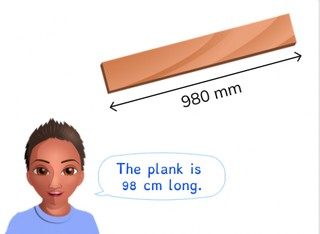Converting mm and cm

# Converting mm and cm

Students learn to convert mm and cm.

No account needed.8,000 schools use Gynzy92,000 teachers use Gynzy1,600,000 students use Gynzy

## General

The students learn to convert millimeters to centimeters and vice versa.

## Standards

CCSS.Math.Content.4.MD.A.1

## Learning objective

Students will be able to convert millimeters (mm) to centimeters (cm) and vice versa.

## Introduction

On the interactive whiteboard there are four images with a number. Next to this are the measurements mm, cm, dm, and m. The students determine which units of length belong which each image. Discuss which units of measurement belong with the images and why the students think this. You can drag the units to the correct places.

## Instruction

You explain, using a diagram of the metric system, that when you want to convert millimeters to centimeters, you take one jump to the left. You state that you divide the millimeters by ten, because you are taking one jump to divide by ten. You show this by converting several measurements in millimeters to centimeters. After this you explain that when you don't have an exact number of centimeters, you will have some millimeters left over. You do this by first determining how many centimeters you have, and then seeing how many millimeters you have left over. You have the students drag the numbers to the correct boxes. Next you explain that when you want to convert centimeters to millimeters, you take one jump to the right an so you multiply by ten. You show this by converting several measurements in centimeters to millimeters. Then you explain that when you don't have an exact number of centimeters, you first convert the centimeters to millimeters and then add on the extra millimeters. The students drag the numbers to the correct places again. Finally you have the students solve the story problems. For this, discuss what steps you must follow when solving this type of story problem.

To check whether students can convert millimeters to centimeters and vice versa, you can ask the following questions:
- How many millimeters is one centimeter?
- What do you do when you convert millimeters to centimeters?
- What do you do when you convert centimeters to millimeters?

## Quiz

The students test their understanding of converting millimeters and centimeters through ten exercises. For some of the exercises they must convert from millimeters to centimeters, and in the others they must convert from centimeters to millimeters. Some of the exercises are story problems.

## Closing

Repeat the learning goal and its importance. Next, there are several amounts of millimeters converted to centimeters on the interactive whiteboard. In each conversion there is a splotch of paint that is covering up a number. Have the students determine what number or unit of length is behind each of the splotches of paint. By erasing them, you can see the answer underneath.

## Teaching tips

When students have difficulty with this learning goal, you can give them a diagram of the metric system, in which you only have centimeters and millimeters with an arrow for ÷ 10 and an arrow for ×10.

## Instruction materials

You may print out a diagram of the metric system.

### The online teaching platform for interactive whiteboards and displays in schools

• Save time building lessons

• Manage the classroom more efficiently

• Increase student engagement